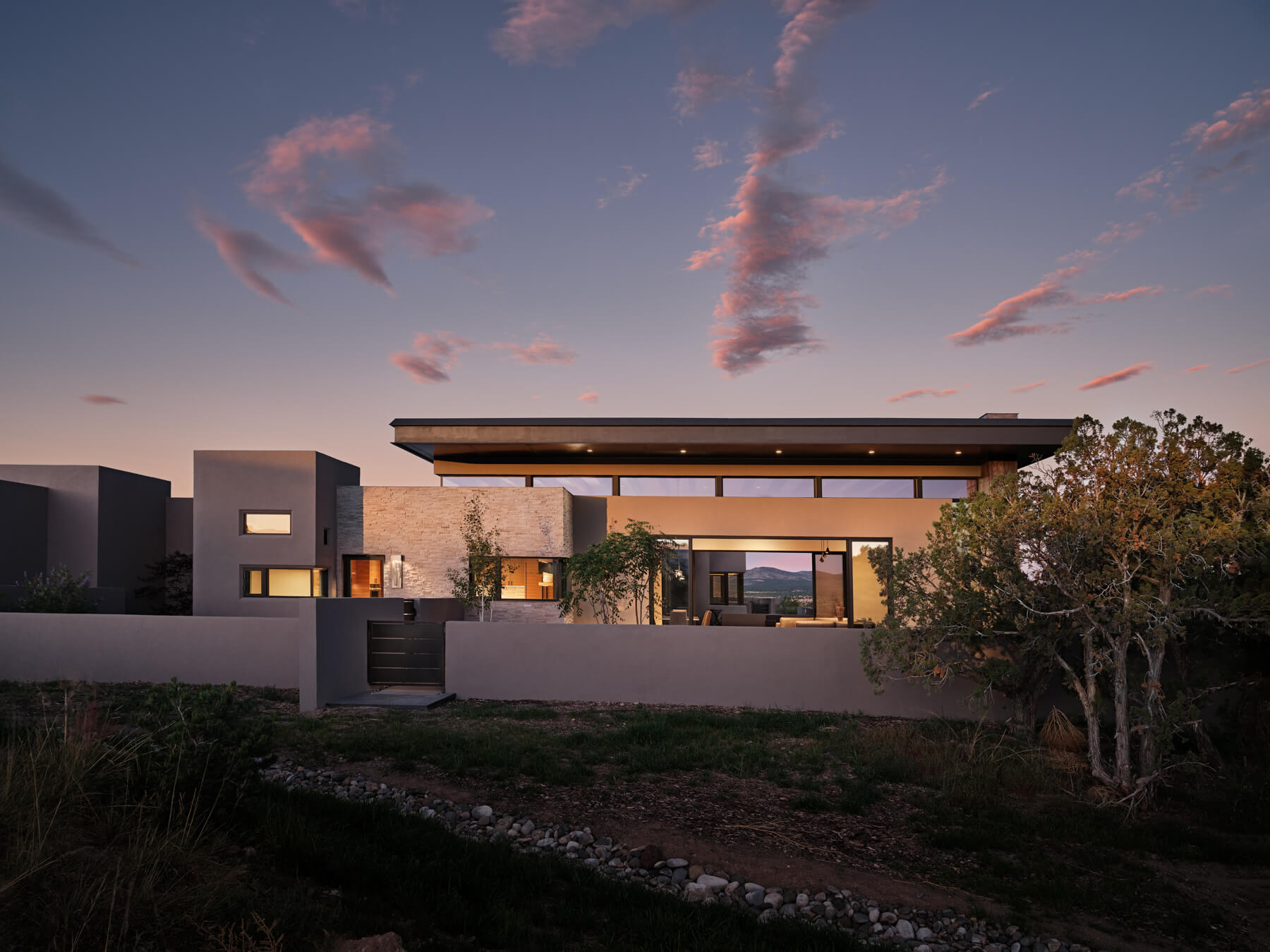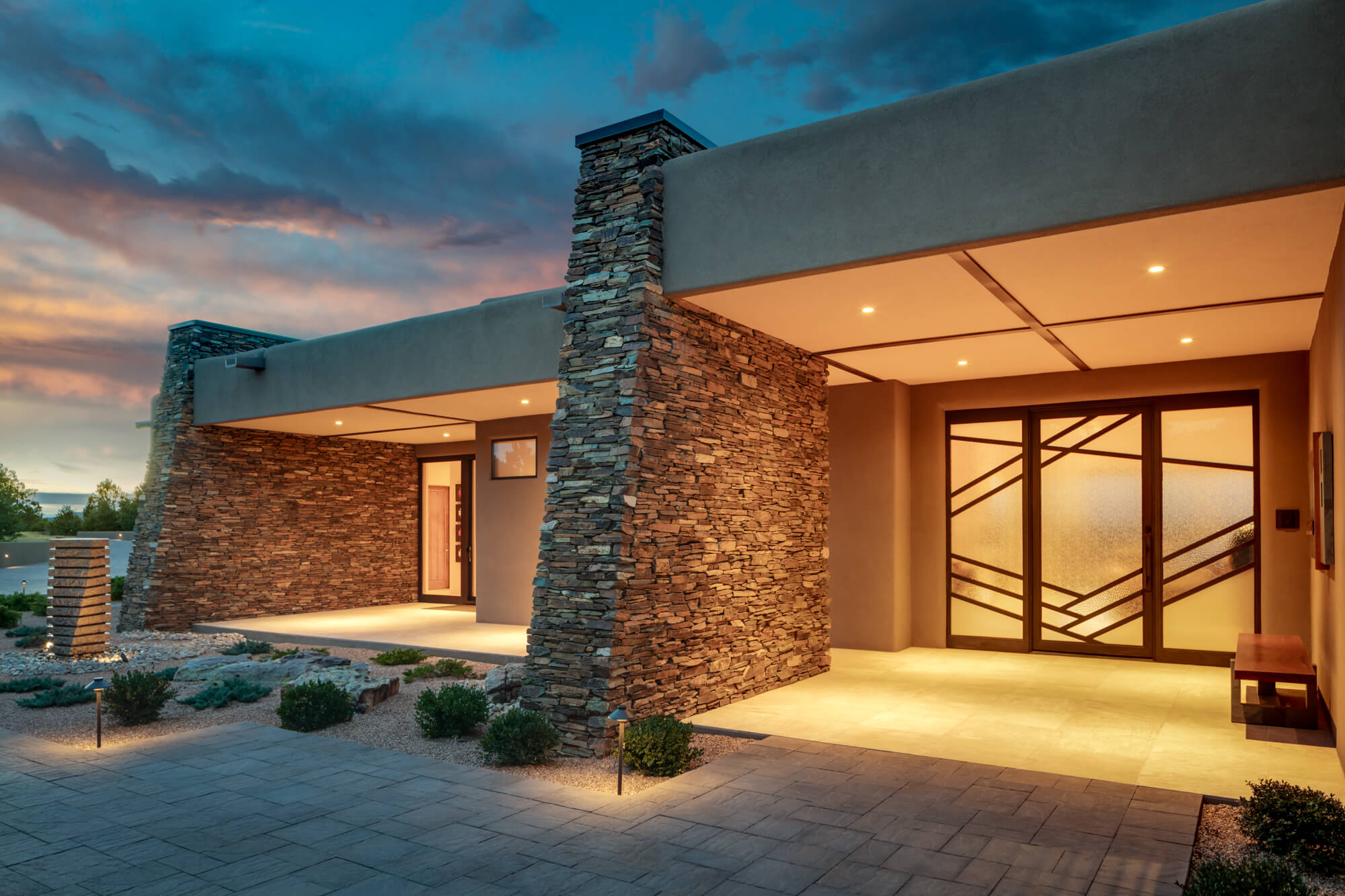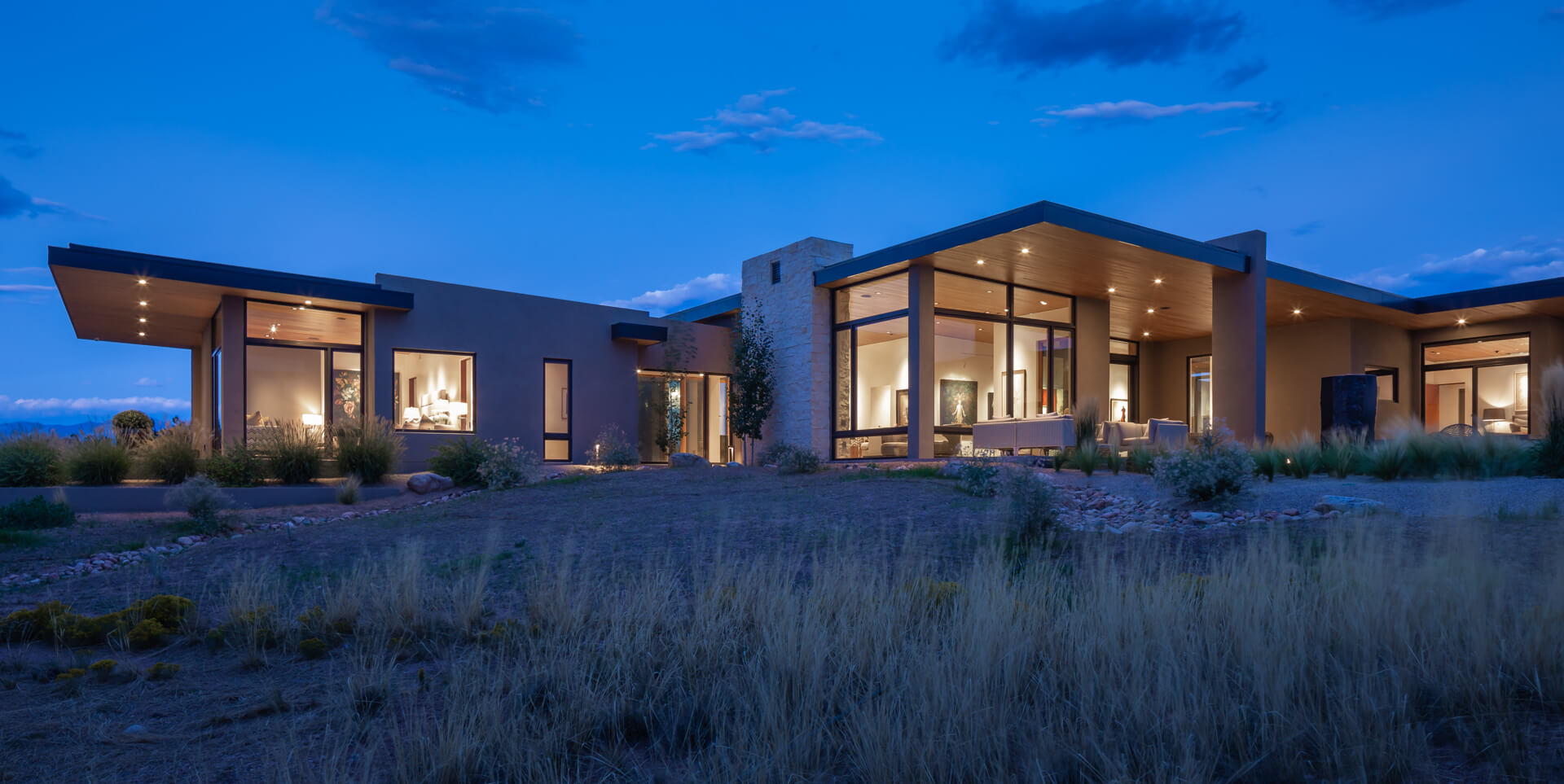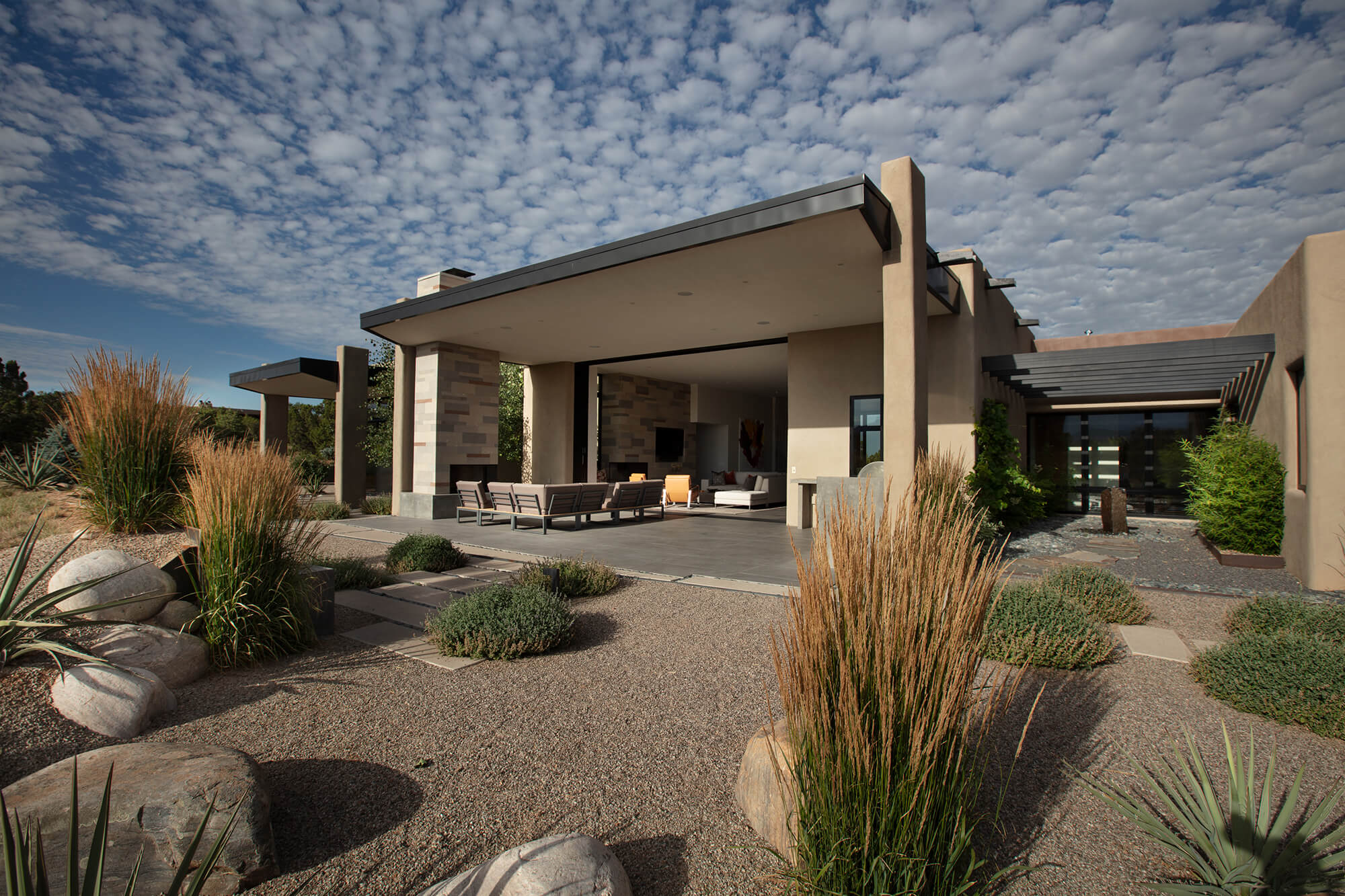# Home“Elsewhere the sky is the roof of the world;
here, the earth is the floor of the sky.”“Elsewhere the sky is the roof of the world;
here, the earth is the floor of the sky.”## Serenity

“Elsewhere the sky is the roof of the world;
here, the earth is the floor of the sky.”## Sanctuary

“Elsewhere the sky is the roof of the world;
here, the earth is the floor of the sky.”## Dreamscape

“Elsewhere the sky is the roof of the world;
here, the earth is the floor of the sky.”## Refuge

“Elsewhere the sky is the roof of the world;
here, the earth is the floor of the sky.”## Tranquility

“Elsewhere the sky is the roof of the world;
here, the earth is the floor of the sky.”## Splendor

“Elsewhere the sky is the roof of the world;
here, the earth is the floor of the sky.”## Captivating

“Elsewhere the sky is the roof of the world;
here, the earth is the floor of the sky.”

“Elsewhere the sky is the roof of the world;
here, the earth is the floor of the sky.”